Vibrations Index Page

Natural Frequencies to Traverse Vibrations

Introduction

The information below relates to natrural frequency of traverse vibration.     The units for the various parameters must be consistent. i.e for metric calculations length is m, force = N, mass = kg. etc.

It can be shown that the critical whirling speed for a shaft is equal to the fundamental frequency of transverse vibration.

Background notes showing how the natural frequencies are derived and the relationship to the shaft whirling velcities are found at Derivation of Natural Frequencies

For members of excelcalcs.com I have created a set of spreadsheet calcs based on the equation below ref. ExcelCalcs.com calculation Shafts -Natural Frequencies / Whirling speeds

Nomenclature

The units below relate to application of the equations using SI metric values

 L = Length (m) m = mass/Unit length (kg/m) M = Mass of Disk (kg) E = Youngs Modulus (N/m2 f = frequency of vibration /whirling frequency (Hz= cycles/s) g= acceleration due to gravity (m/s 2) I = Area Moment of Inertia (m4) G = Torsional Modulus (N.m-2) s = Shaft torsional stiffness = GJa/L (Nm.rad) J = Shaft polar moment of inertia (m4) JM1, JM2,JM3...= polar moment of inertia of disc mass = MX.k2 (X= 1,2,3...)(kg.m2) k = radius of gyration of disc (m) y = deflection (m)

It can be shown that the critical whirling speed for a shaft is equal to the fundamental frequency of transverse vibration.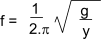g = accelaration due to gravity (9.81 m/s2 ). y = static deflection at mass

The values for natural frequencies relate to cycle/unit time.  The higher harmonic modes are not listed.  To obtain values for wn in radians/s multiply values by 2.p.

Fundamental Natural Frequencies of Beams (bending) and Shafts (Torsion)

Cantilevered Beam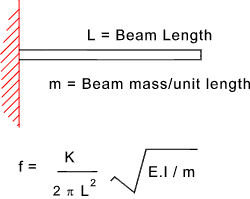Mode 1 2 3 4 K 3,52 22,0 61,7 121

Note: if equation used for whirling speed assume rotating member is supported using "a long bearing /bearing proving substantial angular support e.g. needle bearings"

Simply Supported Beam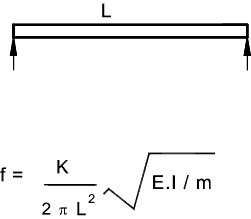Mode 1 2 3 4 K 9,87 39,5 88,8 158

Note: if equation used for whirling speed assume rotating member is supported using "short bearings providing little angular restraint e.g a spherical ball bearing"

Beam with fixed ends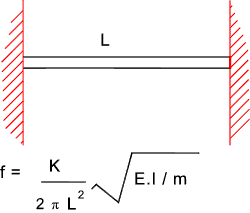Mode 1 2 3 4 K 22,4 61,7 121,0 200

Note: if equation used for whirling speed assume rotating member is supported using "a long bearing /bearing proving substantial angular support e.g. needle bearings"

Beam with one end fixed and one end Simply Supported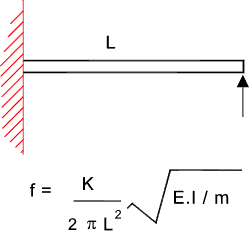Mode 1 2 3 4 K 15,4 50 104 178

Note: if equation used for whirling speed assume rotating member is supported using "a long bearing /bearing proving substantial angular support e.g. needle bearings" and a short bearing providing little angular support e.g a spherical ball bearing

Mass on Cantilever beam

Note: Assume beam of negligible mass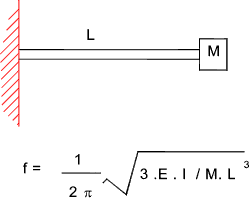Note: if equation used for whirling speed assume rotating member is supported using "a long bearing /bearing proving substantial angular support e.g. needle bearings"

Central mass on Simply supported beam

Note: Assume beam of negligible mass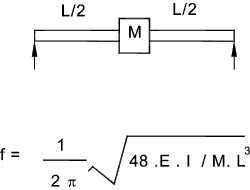Note: if equation used for whirling speed assume rotating member is supported using "short bearings providing little angular restraint e.g a spherical ball bearing"

Mass on Simply supported beam. Off-Centre

Note: Assume beam of negligible mass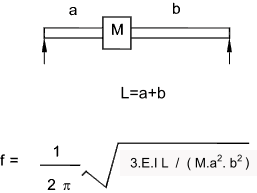Note: if equation used for whirling speed assume rotating member is supported using "short bearings providing little angular restraint e.g a spherical ball bearing"

Mass on beam fixed at bot ends. Mass central

Note: Assume beam of negligible mass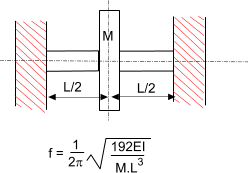Note: if equation used for whirling speed assume rotating member is supported using "longt bearings providing full angular restraint e.g needle bearings"

Multiple Mass on beam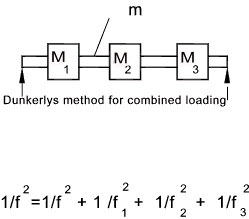Note: if equation used for whirling speed assume rotating member is supported using "short bearings providing little angular restraint e.g a spherical ball bearing"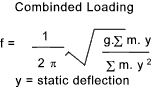Mass on Cantilevered Shaft In Torsion

The following two examples identify the natural torsional frequency of shafts with heavy disks on the ends.

Note: Assume shaft of negligible mass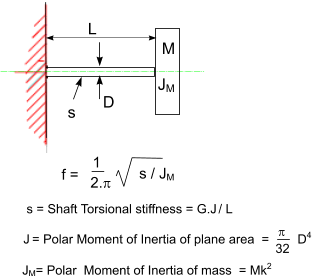Shaft with mass at both ends

Note: Assume shaft of negligible mass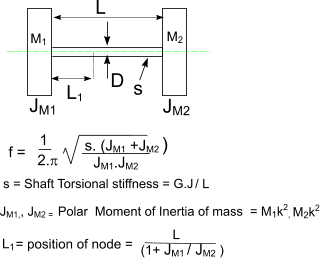Whirling of shafts

When a shaft rotates at a speed equal to the natural frequency of traverse vibration, it results in vibratons at this frequency which becomes large and this is identified as shaft whirling.     This also occurs at multiples of the resonant frequency......It must be noted that a Perfectly balanced shaft will not whirl at any speed

Additional notes on this topic are found at Natural Frequencies to Traverse Vibrations- Whirling

 Links to shaft Vibrations Tutorial - Forced Vibrations ...A very useful set of notes on the subject. EAR_Online ...E A R Speciality Composites- Including Useful Article Taylor Devices ... Taylor Devices Free Techical Papers Literature on Vibration, Shock, Damping, Isolation.. Euro Bearings ...Product information on Vibrations Dampers. Noisenet ...Some interesting and easy to follow notes- Sponsered by Martex Basic Theory of Vibrations...Chapter two of a text book by RE Blake
Vibrations Index Page